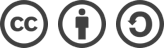## SDUT 2059 简单n!（大数阶乘）解题报告

2016-01-26 1,528 次浏览

## 题面

Time Limit: 1000MS Memory limit: 65536K

1 4

0! = 1 1! = 1

0! = 1 1! = 1 2! = 2 3! = 6 4! = 24

## 解题思路

_36230
x____8
289840

#include <stdio.h>

int main(int argc, char const *argv[])
{
//数组a的大小能存下150!(200多位的数)即可
//len表示当前大数的长度
//carry表示进位数
//product表示当前位做乘法运算后的乘积
int a, n, len, carry, product, i, j;
while(~ scanf("%d", &n))
{
printf("0! = 1\n");
len = 1;
a = 1;
for(i=1; i<=n; ++i)
{
carry = 0;
for(j=0; j<len; ++j)
{
//计算当前位的乘积
product = a[j]*i + carry;
//进位后的值通过乘积取余10000得到
a[j] = product % 10000;
//进位数通过乘积/10000得到
carry = product / 10000;
}
a[j] = carry;
//计算完毕后如果存在进位，则长度+1
if(carry) len++;
printf("%d! = %d", i, a[len-1]);
for(j=len-2; j>=0; --j)
{
//不足四位数的前补0来输出
printf("%04d", a[j]);
}
printf("\n");
}
printf("\n");
}

return 0;
}

## 其他解题方法

import java.util.*;
import java.math.*;

public class Main {
public static void main(String[] args) {
Scanner in = new Scanner(System.in);
BigInteger a;
int n;
while(in.hasNext()) {
n = in.nextInt();
a = BigInteger.ONE;
System.out.println(0 + "! = " + 1);
for(int i=1; i<=n; ++i) {
a = a.multiply(BigInteger.valueOf(i));
System.out.println(i + "! = " + a);
}
System.out.println();
}
}
}bLue 创作，采用 知识共享署名 3.0，可自由转载、引用，但需署名作者且注明文章出处。